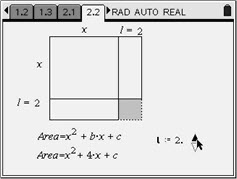# Activities

••• ##### Author9-12

40 Minutes

• ##### Device
• TI-Nspire™
• TI-Nspire™ CAS
• ##### Software

TI-Nspire™
TI-Nspire™ CAS

1.7

## Area of the Missing Square#### Activity Overview

In this activity, students will be introduced to an area model for representing a quadratic equation. Students will explore the relationship between the value of b and c, in y = x2 + bx + c, form of the quadratic equation. The relationship will be examined with integer and non-integer values in order to help students recognize a pattern. Students will then apply their knowledge by answering several questions using the relationship.

#### Key Steps

•In this problem, students will use a slider to increase the length of the square in increments of one. They will find the area of the square needed to complete the square. Students should observe the relationship between the coefficient of x and c.